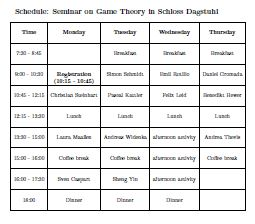# September : Game Theory

#### Date

Monday, September 10th, to Thursday, September 13th

#### Location

Schloss Dagstuhl,

see Dagstuhl’s Program

#### Organizers

Simon Schmidt und Andrea Thevis (Saarbrücken)

Game Theory

#### Participants

Name
Benedikt Hewer
Pascal Kattler
Felix Leid
Laura Maaßen
Emil Rotilio
Simon Schmidt
Ricardo Schnur
Christian Steinhart
Andrea Thevis
Andreas Widenka
Sheng Yin

#### Schedule#### Description of the talks

TitleDescriptionLiteratureReferee
TAKE-AWAY-GAMES AND THE GAME OF NIMThis talk introduces basic definitions and concepts which are important for
other talks (e.g., perfect information, combinatorial, impartial, partizan game). We then
study different types of games: Take-Away-Games can be analyzed with the help of P- and
N-positions and backward induction. (Also mention subtraction games which are a generalization
of Take-Away-Games.) The Nim-sumis used to study the Game of Nim.
Introduction, Chapter I Section 1 and 2 of [Fer]Christian Steinhart
GRAPH GAMES AND SUMS OF COMBINATORIAL GAMESIn this talk we define directed graphs and use themto describe and study combinatorial
games. We then introduce the Spargue-Grundy function. This function can be
used to analyze combinatorial game. (show this on an example)
In the second part of the talk, we define the sum of graph games. We discuss the Spargue-
Grundy theorem and its applications.
Chapter I Section 3 and 4 of [Fer]Laura Maassen
COIN TURNING GAMESThe goal of this talk is to introduce and study Coin Turning Games.Chapter I Section 5 of [Fer]
GREEN HACKENBUSHIn this talk we study the game Green Hackenbush (see [Fer]). For this, we use
concepts introduced in the previous talks, e.g. the Spargue-Grundy function. If time permits,
we discuss a generalization called Blue-Red Hackenbush (see [BCG]).
Chapter I Section 6 of [Fer] and [BCG]Sven Caspart
STRATEGIC FORM AND MATRIX GAMESIn this talk, we introduce the strategic form of a two-person zero-sum game
and state theMinimax Theorem, which will also appear in other talks.
Finite two-person zero-sum games are also called matrix games and in the second part of
this talk we discuss 2x2, 2xn and mx2 matrix games as well as Latin square games.
Chapter II Section 1 and 2 of [Fer]Simon Schmidt
PRINCIPLE OF INDIFFERENCEWe first state the Equilibrium Theorem in this talk, which leads us to the Principle
of Indifference. We use this principle to solve games like the diagonal, triangular and
symmetric games. Then we introduce the concept of Invariance and consider Colonel Blotto
games (a class of tactical military games) as example.
Chapter II Section 3 of [Fer]Pascal Kattler
SOLVING FINITE GAMESThis talk introduces the Bayes strategy, also called best response. We express
theMinimax Theorem more simple and prove it by reducing it to a linear programming problem.
Then we describe the PivotMethod for solving games and give an example.
Chapter II Section 4 of [Fer]Andreas Widenka
EXTENSIVE FORMIn this talk we introduce the extensive form of a two-person zero-sum game,
modelled by a directed graph. We show that one can represent strategic formgames in extensive
formand reduce a game in extensive formto a game in strategic form. We look at games
of perfect information which are games in extensive formand behavioral strategies which are
useful for such games.
Chapter II Section 5 of [Fer]Sheng Yin
RECURSIVE AND STOCHASTIC GAMESWe start with defining matrix gameswith games as components and look at Decomposition
of such games. Then we discuss multistage games and define recursive games.
There are \epsilon-optimal strategies for such games.
The second part of the talk concerns stochastic games. We define those games and look at
the Shapley iteration which approximates the solution.
Chapter II Section 6 of [Fer]Emil Rotilio
INFINITE GAMESThe first part of this talk deals with semi-finite games, where we state theMinimax
theorem and look at solutions of such games.
After that, we discuss continuous games, concave and convex games. If time permits, we
compare two uniform[0,1] pokermodels.
Chapter II Section 7 of [Fer]Felix Leid
BIMATRIX GAMES AND NONCOOPERATIVE GAMESThis talk introduces the strategic form and the extensive form of two-person
general-sumgames. Bimatrix games are finite two-person general-sum games.
The second part of this talk deals with noncooperative games. We will see that every finite
n-person game has at least one strategic equilibrium, also known as Nash equilibrium.
Chapter III Section 1 and 2 of [Fer]Daniel Gromada
MODELS OF DUOPOLYIn this talk, we look at different models of Duopoly (two sellers/firms in one
market), namely the Cournotmodel, the Bertrand model and the Stackelberg model.
Chapter III Section 3 of [Fer]Benedikt Hewer
COOPERATIVE GAMESThis talk concerns cooperative games, where we distiguish between cooperative
games with transferable utility and those with non-transferable utility. The latter can be
approached through the Nash Bargaining Model. Another approach is the Lambda-Transfer
due to Shapley.
Chapter III Section 4 of [Fer]Andrea Thevis
GAMES IN COALITION FORMThe goal of this talk is to analyze in which situations different players should
forma coalition and how to distribute the profit.
After giving introducing formal definitions of games in coalition form and characteristic
functions, we explain how a game in coalition form can be transformed into strategic form
and vice versa. We then discuss the distribution of the profit won while playing a game in a
coalition. Finally, we discuss the power of individual players in a coalition.
Chapter IV Section 1 to 3 of [Fer]

#### Literature

[Fer] Game Theory by Thomas S. Ferguson

[BCG] Winning Ways for your Mathematical Plays by E.R. Berlekamp, J.H. Conway, and R.K. Guy